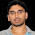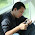Saturday, June 16, 2012

Contours - 3 : Extraction

Hi,

This is our third article on contours and direct continuation of Contours 1 : Getting Started and Contours - 2 : Brotherhood. Hope you have read and understood it well before reading this.

In this article, we won't be using any new function from OpenCV, instead we use the methods from previous article to extract useful data of a contour or an object. You will be using some of these routines in your codes often. So we can get into the topic now.

What are these features actually ? Yes, that is a relative question, i think. It can be anything you want to find about an object and it directly depends on your goals. Some times, you may be interested in its size, sometimes its center, or its average color, or minimum and maximum intensity of that object, and even its orientation, ie its slope etc. I would like to list some of the normally used features.

1 - Area and Perimeter :

This, we have already discussed in last articles, which can be found out using cv2.contourArea() and cv2.arcLength() functions, respectively. You can refer that.

2 - Centroid :

Centroids are found using cv2.Moments() function where centroid can be defined as :

centroid_x = M10/M00 and centroid_y = M01/M00

M = cv2.moments(cnt)
centroid_x = int(M['m10']/M['m00'])
centroid_y = int(M['m01']/M['m00'])Remember, actual result obtained will be 'float', so convert it into 'int'.

If you draw a circle at that point, you can see the centroid.

3 - Aspect Ratio :

Aspect Ratio is the ratio of width to height.

It will be useful in the cases where you want to filter out some shapes. The best example which comes to my mind is ANPR (Automatic Number Plate Recognition). ANPR is used in several traffic surveillance systems to track vehicles going that way. So, in such scenarios, first step is to extract rectangles in the image (since number plate is a rectangle). But there may be false ones also. So use aspect ratio to remove unwanted rectangles (You can google several papers using this method)

x,y,w,h = cv2.boundingRect(cnt)
aspect_ratio = float(w)/h


4 - Extent :

Extent is the ratio of contour area to bounding rectangle area.

area = cv2.contourArea(cnt)
x,y,w,h = cv2.boundingRect(cnt)
rect_area = w*h
extent = float(area)/rect_area


5 - Solidity :

Solidity is the ratio of contour area to its convex hull area.

area = cv2.contourArea(cnt)
hull = cv2.convexHull(cnt)
hull_area = cv2.contourArea(hull)
solidity = float(area)/hull_area


6 - Equivalent Diameter :

Equivalent Diameter is the diameter of the circle whose area is same as the contour area.

It is calculated as, Equivalent Diameter =  4 * A / Π
where A = Area of contour

area = cv2.contourArea(cnt)
equi_diameter = np.sqrt(4*area/np.pi)


7 - Orientation :

Orientation is the angle at which object is directed.

(x,y),(MA,ma),angle = cv2.fitEllipse(cnt)


8 - Pixel Points :

In some cases, we may need all the points which comprises that object. It can be done as follows:

mask = np.zeros(imgray.shape,np.uint8)


9 - Maximum Value and Minimum Value :

We can find these parameters using a mask image.

min_val, max_val, min_loc,max_loc = cv2.minMaxLoc(imgray,mask = mask)


where mask is same as above. Remember, this is for grayscale images, not for color images.

10 - Mean Color or Mean Intensity :

Here, we can find the average color of an object. Or it can be average intensity of the object in grayscale mode. We again use the same mask to do it.

mean_val = cv2.mean(im,mask = mask)


Remember, if you are trying for color matching or color based object tracking, first convert image to HSV space, because HSV is more better representation of color that RGB space. We will deal it in more detail in another article.

11 - Extreme Points :

Extreme Points means topmost,bottommost,rightmost,leftmost points of the object.

leftmost = tuple(cnt[cnt[:,:,0].argmin()])
rightmost = tuple(cnt[cnt[:,:,0].argmax()])
topmost = tuple(cnt[cnt[:,:,1].argmin()])
bottommost = tuple(cnt[cnt[:,:,1].argmax()])


For eg, if I apply it to an Indian map, I get the following result :Extreme Points

For those who couldn't understand above piece of code, I will explain one for you, ie leftmost.

We have contour points (x,y) stored as a [rows,1,2]. Number of rows equal to number of contour points. So to find the leftmost point, we need to find the point where 'x' is minimum. 'y' doesn't matter. So for that, we extract 'x' coordinates of all the points.

x = cnt[ : , : , 0]


Now we find the location of minimum value in it.( Not minimum value, but position or index of the minimum value)

x_min_loc = x.argmin()


Now we find the point (x,y) in cnt at this location(x_min_loc).

point = cnt[x_min_loc]


Sometimes, there may be more than one leftmost points, like rectangles. So we have to take only one of them. And convert it into tuple.

leftmost = tuple(point)


So these are some of the features used frequently.

Now only few more things are there to explain about the contours like convexity defects, point polygon test etc. We will be dealing it in next article.

Regards,
ARK

1.How can i set up the x coord. and find the y value?
Thank you so much!!!

2.Thank you for very usefull articles about contours. Could you suggest how can the nearest countours be merged into the one?

1.Hi, I don't have a good idea for that, although I am looking for such an algorithm somewhere. Please share it if you find it.

3.Can you please tell me what is np, as I am on Java and need to find the equivilant class;

1.'np' is the Numpy library imported as 'np', a shortcut to address Numpy library. It is a Python feature.

>>> import numpy as np

2.Thank you sir, I really appreciate your hard devoted work. I wish I could donate :)

4.Hi, abid rahman do you have c++ version of Extreme Points code?

1.I am sorry, I don't have. But I don't think it will be difficult to implement. So try yourself and let me know if you have any questions.

5.Thanks a lot, Abid. Really nice article